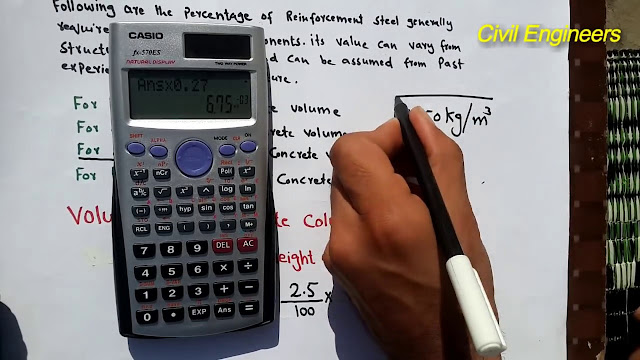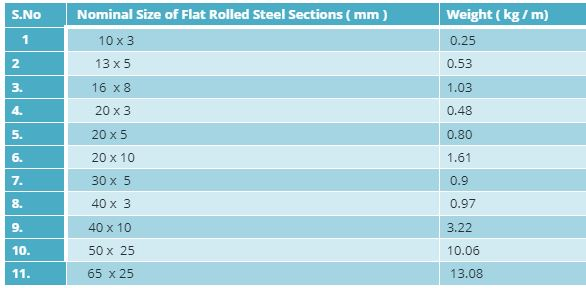How to Calculate the Weight of Steel Rolled Section / Flat Section in Kg/m

# How to Calculate the Weight of Steel Rolled Section / Flat Section in Kg/mSteel is material which is used for making the steel structure. Rolled steel sections area available in the market in various forms such as Angles Sections, Channel Sections, Beam sections, I- sections, T-sections, square Bars, Plates, Flat rolled steel sections etc.
Here is the table that consist different forms of rolled steel sections and their corresponding weight.All the section given above table and their weight in kg /m is taken taken from IS – 800.
Procedure to calculate the weight of Rolled Steel Section is:
1.Calculate the Volume of the section. Consider the length 1 m and rest of the data is mentioned above table.

2. For example in Channel sections
• Divide the sections in three parts. Accordingly calculate the volume of these three parts And add the all these volume.
• Take the density of steel 7850 kg / m3.
•  Weight of section in kg / m is equal to total volume x density of steel.

How to calculate the weight of flat steel rolled sections Flat Steel

Rolled steel Section are available in the size of the range from 10 x 3 to 300 x 25 mm. The standard length of flat sections is 6 meters.

Let us calculate the Weight of Flat steel rolled section is kg per meter.
Here the size of steel Section is 300 x 20
Where:
Width (W) = 300 mm
Thickness (in mm) = 20 mm

So Weight of Flat sections in kg / m = [Width (mm) x thickness (mm)] / 123
Therefore, Weight of flat sections 300 x 20   = (300 x 20) / 123
= 48.78 kg/ m.
Following are the ready made table having nominal size ( mm) and their weight in kg / mSource: civilfieldwork